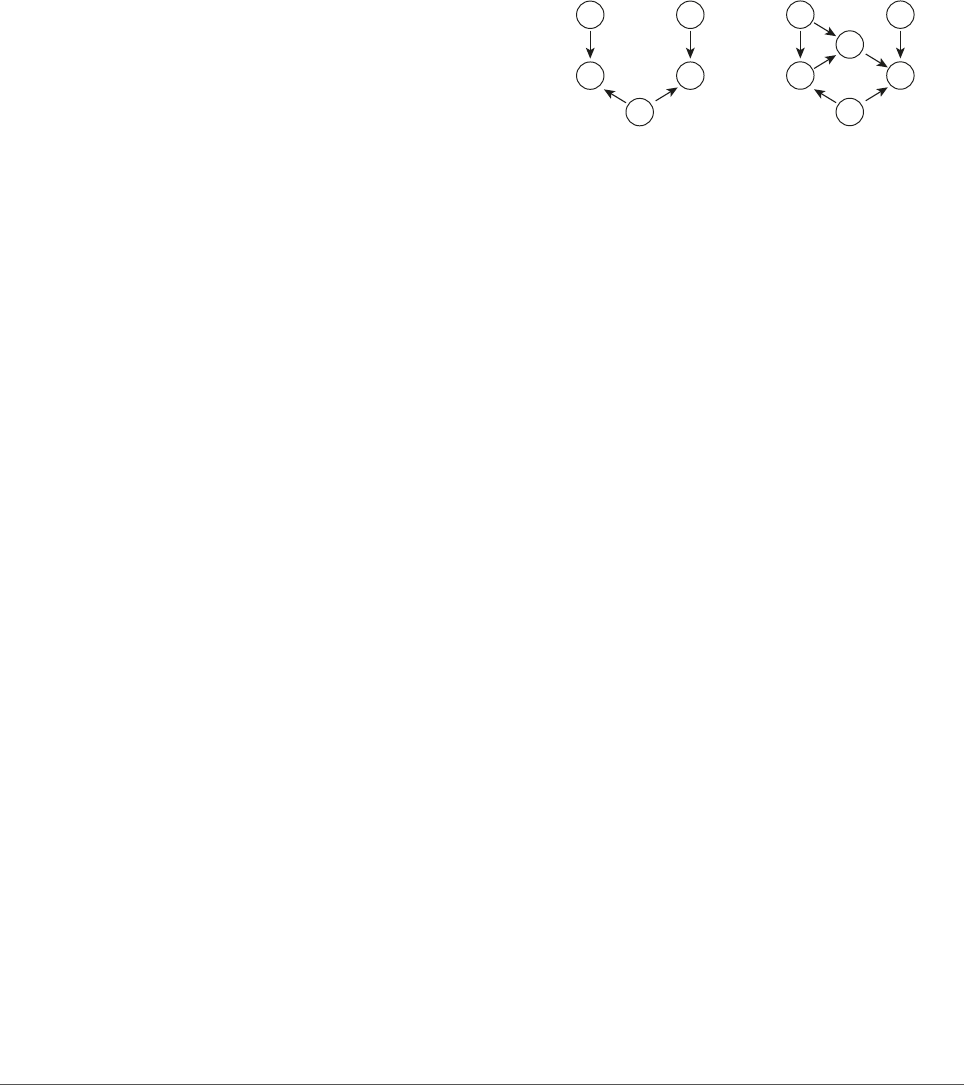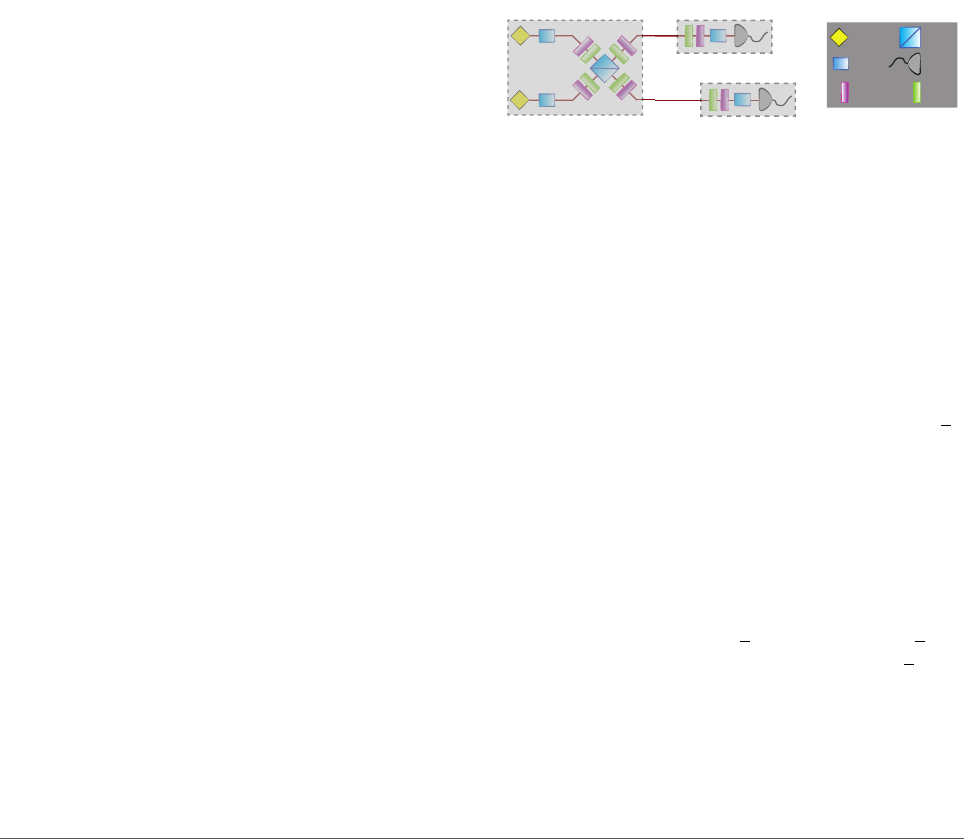Probing the Non-Classicality of Temporal Correlations
Martin Ringbauer
1 2 3
and Rafael Chaves
4
1
Centre for Engineered Quantum Systems, School of Mathematics and Physics, University of Queensland, Brisbane, QLD 4072, Australia
2
Centre for Quantum Computer and Communication Technology, School of Mathematics and Physics, University of Queensland, Brisbane,
QLD 4072, Australia
3
Institute of Photonics and Quantum Sciences, School of Engineering and Physical Sciences, Heriot-Watt University, Edinburgh EH14
4AS, UK
4
International Institute of Physics, Federal University of Rio Grande do Norte, 59070-405 Natal, Brazil
November 15, 2017
Correlations between spacelike separated
measurements on entangled quantum systems
are stronger than any classical correlations and
are at the heart of numerous quantum tech-
nologies. In practice, however, spacelike sepa-
ration is often not guaranteed and we typically
face situations where measurements have an
underlying time order. Here we aim to pro-
vide a fair comparison of classical and quan-
tum models of temporal correlations on a sin-
gle particle, as well as timelike-separated cor-
relations on multiple particles. We use a causal
modeling approach to show, in theory and
experiment, that quantum correlations out-
perform their classical counterpart when al-
lowed equal, but limited communication re-
sources. This provides a clearer picture of the
role of quantum correlations in timelike sepa-
rated scenarios, which play an important role
in foundational and practical aspects of quan-
tum information processing.
Quantum predictions are fundamentally incompat-
ible with the intuitive notion of cause and eﬀect that
underpins all of classical empirical science, as well
as everyday experience. Most famously, Bell’s the-
orem  shows that the correlations revealed by mea-
surements on distant parts of entangled quantum sys-
tems cannot be explained in causal terms, under the
assumptions that local measurement outcomes are not
inﬂuenced by spacelike separated events—local causal-
ity—and that measurement settings can be chosen
freely. This phenomenon has become known as quan-
tum nonlocality, and has been extensively studied for
variants of the scenario above, where spacelike sepa-
rated parties perform measurements on a composite
quantum system .
Yet, in practice we often face situations where
spacelike separation between observers is not guar-
anteed and instead there is some time order underly-
ing the observed physical events. Indeed, it has been
shown that a single quantum system measured at dif-
ferent points in time exhibits Bell-like correlations [3
8]. However, in such a scenario one cannot appeal to
relativity to rule out communication between the time
steps. This can be problematic, since a classical model
with suﬃcient communication can simulate any cor-
relations. Recent research has thus been devoted to
clarifying to what extent the incompatibility between
classical and quantum descriptions encountered in the
spacelike case carries over to the timelike scenario.
Speciﬁcally, one research direction has been to
study how limited resources such as entropy , mem-
ory  or dimension  can lead to a quan-
tum advantage over classical models. On the other
hand, one can relax the local causality assumption in
Bell’s theorem, aiming to explain quantum correla-
tions by classical models augmented with communi-
cation . However, communication is typically
only allowed for the classical system, leading to an un-
fair comparison between classical and quantum mod-
els, and it remains unclear to what extent these re-
sults hold when equal communication power is given
to quantum mechanics .
Here we use a causal modeling approach to allow
a fair comparison of classical and quantum models
of timelike separated correlations. First, we show
that for two time-ordered spatially separated mea-
surements, augmented with a limited amount of clas-
sical communication, quantum correlations outper-
form their classical counterpart. Second, we show
that, in contrast to spatial Bell-type scenarios ,
there are faithful classical causal models reproducing
all the temporal correlations obtained from a series of
projective quantum measurements on a single quan-
tum system. However, we ﬁnd that non-classical cor-
relations can arise in this scenario, when considering a
slightly weaker classical causal model, that is nonethe-
less strictly stronger than previous results , which
are contained as a special case. Finally we derive Bell-
type inequalities for the above scenarios and demon-
strate that they are violated in a photonic experiment.
1
arXiv:1704.05469v2 [quant-ph] 14 Nov 20171 Causal modeling and timelike Bell-
scenarios
In the following we employ the formalism of Bayesian
networks , which provides a natural framework for
classical causal modeling. A central concept in this
framework is that of a directed acyclic graph (DAG),
which consists of a set of nodes, representing the rel-
evant random variables
1
in the considered situation,
and directed edges, representing the causal relations
between those variables. A set of variables X
1
, . . . , X
n
forms a Bayesian network with respect to some DAG
if and only if the probability distribution p(x
1
, . . . , x
n
)
can be decomposed as
p(x
1
, . . . , x
n
) =
n
Y
i=1
p(x
i
|pa
i
) (1)
where P A
i
stands for the set of graph-theoretical par-
ents of the variable X
i
(i.e. all variables that have a
direct causal inﬂuence over X
i
). Without loss of gen-
erality each variable can be understood as a determin-
istic function of its parents plus local noise U
i
that
supplies potential randomness, x
i
= f
i
(pa
i
, u
i
). This
formalism thus enables a distinction between simple
statistical correlations and actual causation by explic-
itly specifying the underlying mechanism generating
the data.
Here we are interested in DAGs containing so-called
latent variables, which are empirically inaccessible. In
the context of Bell’s theorem  these are also known
as hidden variables. For any set of observed corre-
lations, there are in general many DAGs with hid-
den variables that could have produced these obser-
vations. Among these, causal inference is particularly
interested in those fulﬁlling the conditions of minimal-
ity and faithfulness. Minimality requires that, given
two possible causal models, we choose the simplest
one, capable of generating the smallest set of correla-
tions (including the observed one). In turn, faithful-
ness, requires the causal model to be able to explain
the observed data without resorting to ﬁne-tuning of
the causal-statistical parameters. In other words, any
observed (conditional) independence should be a con-
sequence of the causal structure itself, rather than a
speciﬁc choice of parameters. Faithful (i.e. non-ﬁne-
tuned) models are therefore robust against changes in
the causal parameters and thus the preferred choice.
To illustrate the last point, consider the paradig-
matic causal structure of Bell’s theorem in Fig. 1a.
This structure intuitively reﬂects the causal assump-
tions of Bell’s theorem, leading to the so-called local
hidden-variable (LHV) models. First, the two parties
are assumed to be spacelike separated, such that the
correlations between the measurements outcomes A
1
We adopt the standard convention that uppercase letters
label random variables while their values are denoted in lower
case.
and B can only be mediated via a common source
Λ, implying that p(a, b|x, y, λ) = p(a|x, λ)p(b|y, λ).
Second, it is assumed that the experimenters can
freely choose which observables to measure (repre-
sented by the random variables X and Y ), inde-
pendently of how the system was prepared, that is,
p(x, y, λ) = p(x, y)p(λ). Note that these constraints
implied by the causal model appear at an unobserv-
able level since they explicitly involve the hidden vari-
ables Λ. Yet, they also imply observable constraints
in the form of no-signaling conditions, expressed as
p(a|x, y) = p(a|x) and p(b|x, y) = p(b|y), and Bell
inequalities [1, 29].
A
X
B
Y
Λ
A
X
B
Y
Λ
M
ba
Figure 1: Causal structure underlying Bell’s theorem. (a)
Two observers, Alice and Bob, each have the choice of two
measurements represented by the random variables X and Y ,
respectively. The correlation between their measurement out-
comes, modeled as random variables A and B, respectively,
are mediated solely by a common cause in their past—the
hidden variable Λ. (b) Bell’s causal model augmented with
one-way communication from Alice to Bob. The initial state
of the joint system is speciﬁed by the ontic state Λ. First,
Alice performs a measurement with setting x, obtaining out-
come a. She then sends a message m to Bob, who performs
a measurement with setting y, obtaining outcome b.
While quantum correlations obey the no-signaling
conditions, they violate Bell inequalities [1, 29] and
are thus in conﬂict with the assumptions behind the
causal structure in Fig. 1a. In order to maintain a
classical causal explanation, the model in Fig. 1a must
therefore be augmented with additional resources;
something that can only be done at the cost of in-
troducing ﬁne-tuning . For instance, the causal
structure in Fig. 1b can reproduce all quantum corre-
lations, but at the same time allows, in principle, for
non-local correlations between X and B. Hence, in
order to satisfy the no-signaling condition p(b|x, y) =
p(b|y) the causal parameters must be chosen from a
set of measure zero , a signature of ﬁne-tuning.
Studying such non-local classical models can pro-
vide valuable insights into the relation between clas-
sical and quantum theory, and their applications .
However, at the same time such models lead to an
unfair comparison, since allowing for communication
makes not only classical, but also quantum models
more powerful. In practice, it is more natural to
assume a certain underlying causal structure, and
ask what can be achieved with classical and quan-
tum resources? Bell’s theorem is a particular case
of this broader question, referring to spacelike sep-
arated events. However, there are often situations
2where the events are timelike rather than spacelike
ordered. Examples include central quantum infor-
mation tasks, such as teleportation , superdense
coding , and measurement-based quantum compu-
tation , as well as prepare-and-measure scenarios
, sequential Bell scenarios [33, 34] and a sequence
of measurements on a single quantum system .
2 Non-classicality of timelike correla-
tions augmented by communication
Consider the scenario in Fig. 1b, where two distant
parties, Alice and Bob, share pre-established cor-
relations (represented by Λ) and are allowed one-
way communication (the message M). As shown in
Ref. , a classical model of this form (for a large
enough message M) is enough to reproduce all the
correlations obtainable from local measurements on
two-qubit entangled states as in Fig. 1a, which are
described by
p(a, b|x, y) = Tr
(M
a
x
M
b
y
)ρ
, (2)
where M
a
x
and M
b
y
are measurement operators for Al-
ice and Bob, respectively, and ρ is the density matrix
describing the shared quantum state. If, in contrast,
we impose the causal structure of Fig. 1b also to the
quantum case—that is, Bob’s measurement may de-
pend on Alice’s measurement setting and outcome—
then the set of correlations is described by
p(a, b|x, y) =
X
m
Tr
(M
a
x
M
b
y,m
)ρ
. (3)
Note that Bob’s measurement operator now explicitly
depends on the values of X and A (via the message
M)
2
. Clearly, if there are no restrictions on the di-
mensionality of the message, every distribution of the
form above can be also obtained by the classical hid-
den variable model in Fig. 1b, that is, by a model
respecting the decomposition
p(a, b|x, y) =
X
m,λ
p(λ)p(m|x, a)p(a|x, λ)p(b|y, m, λ).
(4)
In fact, it is enough to choose m = x to reproduce
all possible one-way signalling correlations. To see
this, note that the quantum correlations arising from
Eq. (3) are of <